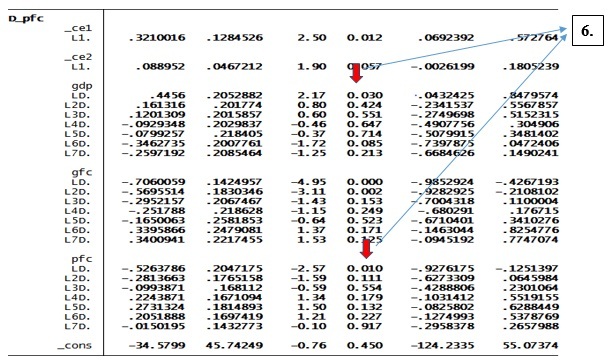# VECM in STATA for two cointegrating equations

By Divya Dhuria & Priya Chetty on September 27, 2018

In the previous article, the Johansen cointegration test revealed the cointegration between time series Gross Domestic Product (GDP), Private Final Consumption (PFC) and Gross Fixed Capital Formation (GFC), containing up to two cointegrating equations. Therefore, unrestricted Vector Auto Regression (VAR) is not applicable in such cases. Vector Error Correction Model (VECM) is a special case of VAR which takes into account the cointegrating relations among the variables.

## Vector Error Correction Model (VECM) in STATA

• Click on ‘Statistics’ in the main tab
• Select ‘Multivariate Time Series’
• Select ‘Vector Error-Correction Model’

The ‘vec’ dialogue box will appear as shown in the figure below. Fill three options; ‘Dependent variables’, ‘Number of co-integrating equations’  and ‘Maximum lag’.

In ‘Dependent variables’ select ‘gdp’, ‘gfc’ and ‘pfc’, using the drop-down menu. In ‘Number of co-integrating equations (rank)’, select ‘2’, since the previous article showed two cointegrating equations using the Johansen cointegration test. Finally in ‘Maximum lag to be included’, select ‘8’, as the previous article showed 8 lags. Click on ‘OK’.

The results will appear as shown in the figure below. Since this case has 8 lags and two equations, the results are lengthy. For more clarity, the explanation is broken down into parts.

## Part I of results of VECM in STATA

This contains the STATA command showing in the result window with information about all the variables as shown in the figure below. For instance, VECM has taken the first difference of these variables, such that they are represented as D_gdp, D_gfc, and D_pfc. Further, the R-square value of all three variables are good enough to justify their causality, and p values close to zero also indicates significance.Figure 4: Part 1 of Results of VECM in STATA

## Part II of VECM in STATA

This part of the result shows the regression equations by taking ‘D_gdp’ as dependent and lagged values of GFC and PFC as independent variables as shown in the figure below. The interpretation is as follows:

1. ‘ce1’ and ‘ce2’ represent two cointegrating equations. To ascertain the long-term causality between GDP and PFC and GFC, the ‘ce1’ and ‘ce2’ have to show a negative coefficient and a significant p-value. As the figure below shows, both the equations do not have negative coefficient but ‘ce2’ has a significant p-value of 0.011. Since all two conditions are missing here, this VECM do not show any long-term causality between GDP and the other two variables PFC and GFC.
2. Furthermore, to examine the short-term causality between variables, see individual lag coefficients and p values for each independent variable (figure below). Thus this part explains the lagged values of GFC and PFC for GDP. As per the result, the only 1st lag of GFC is significant (p-value is 0.00), apart from that no other lag, even of PFC have no significance level. That means the only first lag of GFC has a short-term causality with GDP.Figure 5: Part 2 of results of VECM in STATA

## Part III of VECM in STATA

Similarly, the results of VECM go forward by assuming each of the remaining variables as dependent and others as the independent. For instance, ‘D_gfc’ is dependent and ‘D_pfc’ is independent (figures below).

1. In the case of GFC, a long-term causality from GDP and PFC to GFC is noticeable because ‘ce2’ equation, in this case, has both negative coefficient and significant p-value.
2. A short-term causality is evident only in case of PFC that too on seventh lag with significant p values 0.000.
3. In case of PFC, a long-term causality from GFC and GDP to PFC is absent as both ‘ce1’ and ‘ce2’ equations, in this case, have both non-negative coefficient and insignificant p-value.
4. In the case of PFC, a short-term causality is evident in case of 1st lag of GFC and GDP with significant p values 0.000.Figure 5: Steps 3, 4 and 5 of VECM results in STATAFigure 6: Step 6 of VECM results in STATA
Use 5E3BCCB908B47 to save 6000 on 6001 - 10000 words standard order of research analysis service.

To ensure whether the VECM is correctly specified or not, a set of diagnostics tests such as tests for normality, serial correlation and heteroskedasticity need to be performed. The next article will show how to conduct these diagnostics tests in STATA.